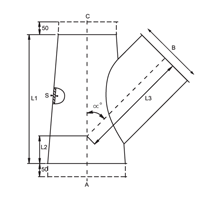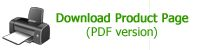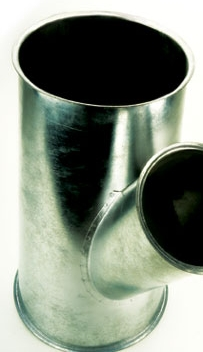### 45° Conical Branch Piece

The principal application of SpeedLock Modular Ducting is any manufacturing industry where fumes, dust or other suspended airborne matter requires extraction without leakage of air or conveyed product, into the immediate atmosphere.

Code BPC45### Dimensional Specifications

 Product Code A mm B mm C mm L1 mm L2 mm L3 mm ∞° S mm BPC45 Choose ID 80 Choose ID 300 calculate calculate 43.80 0.90 BPC45 Choose ID 100 Choose ID 300 calculate calculate 43.80 0.90 BPC45 Choose ID 120 Choose ID 350 calculate calculate 44.00 0.90 BPC45 Choose ID 125 Choose ID 350 calculate calculate 44.00 0.90 BPC45 Choose ID 140 Choose ID 350 calculate calculate 44.10 0.90 BPC45 Choose ID 150 Choose ID 400 calculate calculate 44.20 0.90 BPC45 Choose ID 160 Choose ID 400 calculate calculate 44.20 0.90 BPC45 Choose ID 180 Choose ID 400 calculate calculate 44.30 0.90 BPC45 Choose ID 200 Choose ID 450 calculate calculate 44.40 0.90 BPC45 Choose ID 225 Choose ID 500 calculate calculate 44.50 0.90 BPC45 Choose ID 250 Choose ID 500 calculate calculate 44.50 0.90 BPC45 Choose ID 275 Choose ID 600 calculate calculate 44.60 0.90 BPC45 Choose ID 300 Choose ID 600 calculate calculate 44.60 0.90 BPC45 Choose ID 315 Choose ID 600 calculate calculate 44.60 0.90 BPC45 Choose ID 350 Choose ID 700 calculate calculate 44.70 0.90 BPC45 Choose ID 400 Choose ID 800 calculate calculate 44.70 0.90 BPC45 Choose ID 450 Choose ID 950 calculate calculate 44.70 0.90 BPC45 Choose ID 500 Choose ID 1050 calculate calculate 44.80 0.90 BPC45 Choose ID 550 Choose ID 1150 calculate calculate 44.80 0.90 BPC45 Choose ID 600 Choose ID 1150 calculate calculate 44.80 0.90 BPC45 Choose ID 630 Choose ID 1150 calculate calculate 44.80 0.90 BPC45 Choose ID 650 Choose ID 1150 calculate calculate 44.80 0.90 BPC45 Choose ID 700 Choose ID 1300 calculate calculate 44.80 0.90 BPC45 Choose ID 750 Choose ID 1300 calculate calculate 44.80 0.90 BPC45 Choose ID 800 Choose ID 1450 calculate calculate 44.90 0.90 BPC45 Choose ID 850 Choose ID 1450 calculate calculate 44.90 0.90 BPC45 Choose ID 900 Choose ID 1650 calculate calculate 44.90 0.90

`All product design specifications are subject to change without notice. `
`Images are for illustration purposes only`Material
0.9mm galvanised steel

Sizes
Common sizes are a stock item
Special requirements are manufactured to order

Dimensions
Refer to table - customer to specify A, B, & C

Please note the Branch 'B' determines L1, as in the table below

The maximum diameter reduction between A & C is 200mm. B must however, not be larger then or equal to
(A + C)/2

In the case of double branch pieces, the largest branch determines Length L1developed by Pretty Clever Consulting

### Additional Product Information and Availability

Name

Company

Email

Product Diameter (optional)